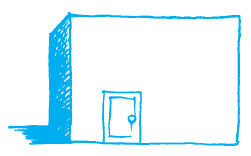# A door of length 2 m and breadth 1 m is fitted in a wall. The length of the wall is 4.5 m and the breadth is 3.6 m (Fig). Find the cost of white washing the wall, if the rate of white washing the wall is ₹ 20 per mm2.Given

From the given details we get to know that

Length of the door = 2 m

Breadth of the door = 1 m

Length of the wall = 4.5 m

Breadth of the wall = 3.6 m

Find out

We have to determine the cost of white washing the wall, if the rate of white washing the wall is ₹ 20 per m2

Solution

Area of the door =Area of rectangle= Length × Breadth

= 2 × 1

= 2 m2

Area of the wall =Area of rectangle= Length × Breadth

= 4.5 × 3.6

= 16.2 m2

Area to be white washed = 16.2 – 2 = 14.2 m2

Cost of white washing 1 m2 area = ₹ 20

Hence cost of whit washing 14.2 m2 area = 14.2 × 20

= ₹ 284

Hence cost of whit washing 14.2 m2 area= ₹ 284(0)(0)